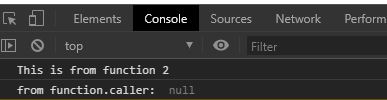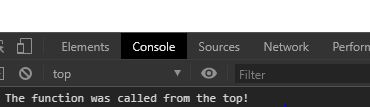# JavaScript function.caller Property

• Last Updated : 02 Dec, 2021

The function.caller property of the JavaScript function object returns the function that invoked the specified function. It returns null if the function “f” was invoked by the top-level code in JavaScript. For functions like strict functions, async functions and generator function this method returns null.

Syntax:

`function.caller`

Parameters: This function does not accept any parameter.

Return Value: This function returns null for strict, async function and generator function callers.

Few examples are given below for a better understanding of the function methods.

Example 1:

## HTML

 ```<``html` `lang``=``"en"``>` `<``head``>``    ``<``meta` `charset``=``"UTF-8"``>``    ``<``meta` `name``=``"viewport"` `content=``        ``"``width``=``device``-width,``        ``initial-scale``=``1``.0">``` `<``body``>``    ``<``script``>` `        ``// Creating func2``        ``function func2() {``            ``console.log("This is from function 2")``        ``}``        ``// Creating func1``        ``function func1() {``            ``func2();``        ``}` `        ``// Call Function 1``        ``func1();` `        ``// null is returned as function``        ``// is strict, async function and``        ``// generator function``        ``console.log("from function.caller: ",``                                ``func1.caller)``    ````` ``

Output:Example 2:

## HTML

 ```<``html` `lang``=``"en"``>` `<``head``>``    ``<``meta` `charset``=``"UTF-8"``>``    ``<``meta` `name``=``"viewport"` `content=``            ``"``width``=``device``-width,``            ``initial-scale``=``1``.0">``` `<``body``>``    ``<``script``>``        ``function myFunc() {``            ``if (myFunc.caller == null)``                ``console.log("The function "``                    ``+ "was called from the top!");``            ``else``                ``console.log("This function's "``                    ``+ "caller was " + myFunc.caller);``    ``}``        ``myFunc()``    ````` ``

Output:Browsers Supported:

• Google Chrome 1 and above
• Microsoft Edge 12 and above
• Mozilla Firefox 1 and above
• Internet Explorer 8 and above
• Safari 3 and above
• Opera 9.6 and above

My Personal Notes arrow_drop_up